# Simple If Statements

### Simple If Statements

The most elementary decision-making statement is the If statement, which has the following general syntax:

` If  Expression1  Then    ' If statement block End If `

The key to the If statement is the logical result of Expression1 . If Expression1 evaluates to logic True , the statements in the If statement block are executed. If the outcome of the test of Expression1 is logic False , the If statement block is bypassed. In that case, program execution resumes with the next program statement following End If .

An example will help you see how the If statement works. Consider the following code fragment:

` If Burglary = True Then   ActivateAlarms()  CallPolice() End If `

The If statement performs a relational test between Burglary and logic True . (You can assume that some earlier code is responsible for setting the Burglary variable to True if someone breaks in to the house.) If the relational test determines that Burglary is logic True , the ActivateAlarms() procedure is called, followed by a call to the CallPolice() procedure. On the other hand, if Burglary is logic False , program execution skips the two procedure calls and proceeds to execute the next program statement following the End If statement.

Figure 11.1 shows how the program flow is executed, depending on the outcome of the relational test on Expression1 .

##### Figure 11.1. Program execution sequence for an If statement.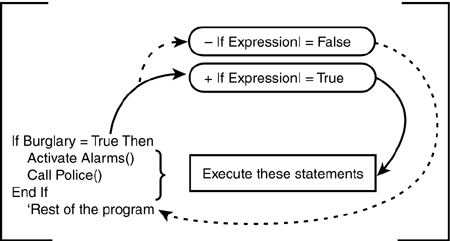In Figure 11.1, when the relational test is False , program flow is diverted around the program lines that are controlled by the If statement block. This means the procedure calls to ActivateAlarms() and CallPolice() are bypassed.

You can write a simple program to show how the If statement works. Start a new project and call it IfElseProject . Add two text boxes, two labels, and two command buttons to the program, as shown in Figure 11.2.

##### Figure 11.2. Placement of objects on the program form.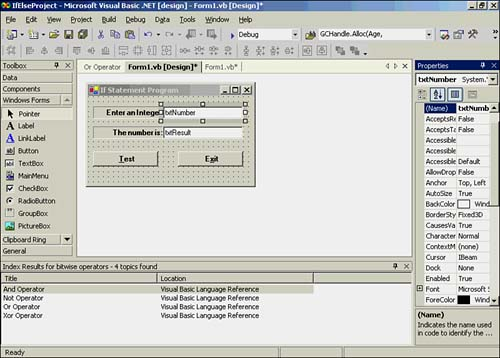Name the Test button btnTest and the Exit button btnExit . In the btnExit object's Click() event, add the call to Me.Dispose() as you usually do.

The only additional code needed for this program is shown in Listing 11.1.

##### Listing 11.1 Code for the btnTest Object's Click() Event
` Private Sub btnTest_Click(ByVal sender As System.Object, ByVal e As _              System.EventArgs) Handles btnTest.Click  Dim Number As Integer  Number = CInt(txtNumber.Text)  txtResult.Text = "Even"  If Number Mod 2 = 1 Then   txtResult.Text = "Odd"  End If End Sub `

When the user clicks the Test button, an integer variable named Number is defined and assigned the number the user enters in the txtNumber text box. Again, you must call CInt() to convert the string in the text box into an integer variable. The program then assigns the string literal "Even" to the txtResult text box.

The If statement tests whether Number is an odd or even number. It performs the test by using the Mod arithmetic operator. Because Mod returns the remainder of integer division, any odd number returns 1 as the result of the Mod expression. If the number is even, Mod returns . The program then performs a relational test to see if the Mod expression returns 1 . If the relational test is True , the txtResult string is changed to "Odd" . If the relational test is False , the assignment statement of "Odd" into the txtResult text box is bypassed, and Even remains displayed.

Let's examine how the processing of the If statement is done if the user enters 10 :

` If Number Mod 2 = 1 Then    If 10 Mod 2 = 1 Then   If 0 = 1 Then `

How does Visual Basic .NET process the second line of this code? Recall from Chapter 10, "Relational and Logical Operators," that arithmetic operators have higher precedence than relational operators. The second line actually behaves as though it were written like this:

` If (10 Mod 2) = 1 Then `

Because 10 divided by 2 is 5, with no remainder, the Mod operator returns . The statement then appears as this:

` If 0 = 1 Then `

This line uses the equality relational operator to see if 0 equals 1, which it does not. The result of the relational test for equality is False , so the statement is reduced to this:

` If 0 Then `

Because Expression1 of the If statement is logic False (that is, ), the If statement block code is not executed, and txtResult remains unchanged, with the string "Even" . It's simple!

#### Using the Visual Basic .NET Debugger to Watch Program Flow

You can use the Visual Basic .NET debugger to see how different values for Number affect the flow of a program. To do this, you need to set a breakpoint on the line where the If statement appears in the program. With the program code showing in the Form window, click in the gray area to the left of the If statement line. Your display should look similar to Figure 11.3.

##### Figure 11.3. Setting a debugger breakpoint on the If statement.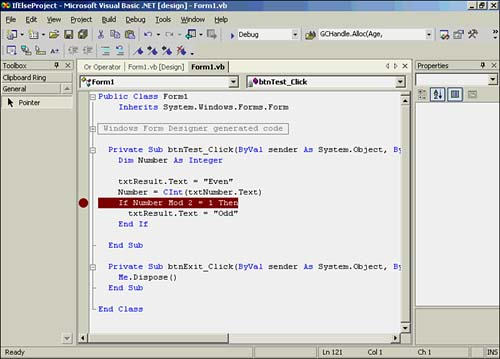Now run the program, enter the value 10 in the txtNumber text box, and click the Test button. Your screen should look similar to the one shown in Figure 11.4.

##### Figure 11.4. Program execution suspended at the breakpoint statement.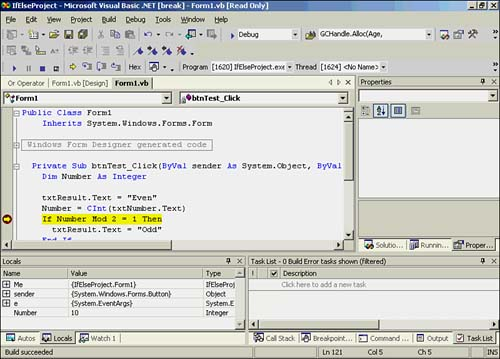As shown in Figure 11.4, Visual Basic .NET suspends program execution at the statement where the breakpoint is set. Notice that the Locals window shows that Number has the value 10 . (If your screen does not show the Locals window, simply click the Locals tab at the bottom of the window.)

You should also notice that the color of the statement line where the breakpoint is changes to yellow. Visual Basic .NET uses this color to show you what line is to be executed next.

Programmer's TipWhen program execution stops at a breakpoint statement, the statement line is shown in yellow. This means that Visual Basic .NET has not yet executed the line shown in yellow. In other words, breakpoints suspend program execution before the breakpoint statement is executed.

##### Single-Stepping

When a program reaches a breakpoint, you can cause Visual Basic .NET to execute the next statement to see which statement is executed next. To do this, you can have the debugger execute one line at a time; this is called single-stepping the program. To single-step the program, you press the F10 key. The F10 key tells Visual Basic .NET: "Hey, Visual Basic .NET! Wake up from your program suspension and execute the next line in the program!"

If you enter 10 (or any other even number) in the txtNumber text box and press the F10 key, you should see the yellow line jump to the End If statement. In other words, the If test is logic False , so the test causes the program to skip the statement in the If statement block (that is, the assignment of "Odd" to txtResult ).

Now you should press the F5 key. The F5 key tells Visual Basic .NET to resume program execution at full speed.

If you want to remove a breakpoint from a statement line, you move the cursor to the edge of the screen and click. This action toggles the breakpoint off. If you click again, the breakpoint is reactivated.

You should try running the program several times, entering odd and even numbers to verify that the program behaves as it should. I encourage you to do this with the breakpoint active so you can single-step through the program and see how it behaves.

#### A Program Simplification

You might have had a nagging feeling in the back of your mind when you saw that the If statement was resolved to this when an even number was entered:

` If 0 Then `

If you did, good! You remembered that in the general format for the If syntax, the value of Expression1 determines whether the If statement block is executed. If that's the case, do you even need the relational test?

What would happen if you replaced this If statement:

` If Number Mod 2 = 1 Then `

with this:

` If Cbool(Number Mod 2) Then `

This second variation of the If statement doesn't contain the relational test for equality. If you change the statement to this new variation and compile and run the program, does it behave exactly as it did before? You should single-step the program to find out.

You should discover that the program functions exactly as it did before. This happens because you can treat the value returned by the Mod operator as logic True or False , depending on the value entered for Number . If the user enters 13 , for example, the expression becomes this:

` If Cbool(13 Mod 2) Then  If 1 Then `

Because 13 divided by 2 is 6, with a remainder of 1, the Mod operator returns 1 . The If statement interprets this remainder as logic True for Expression1 of the If statement, causing the "Odd" string literal to be assigned to txtResult . Remember that an even number produces a Mod value of , and that is viewed as logic False for Expression1 . This causes the If test to bypass the assignment of "Odd" to txtResult .

Programmers love economy of expression (that is, they don't like to type more than they have to). As a result, Expression1 of an If statement is often written without the relational test.

#### Function Calls and If Tests

You can modify your program slightly and write a function that tests for an even or odd number. After all, after you write the function, you can reuse it in other programs if you want. The code for the OddEven() function is shown in Listing 11.2.

##### Listing 11.2 Code for the OddEven() Function
` Public Function OddEven(ByVal Number As Integer) As Integer  ' Purpose: This function returns 1 if the number is odd,  '      and returns 0 otherwise.  '  ' Arguments:  '  Number   The integer number to test  '  ' Return Value:  '  integer   1 if odd, 0 if even  '  OddEven = Number Mod 2 End Function `

In the first line of the function is this statement:

` Public Function OddEven(ByVal Number As Integer) As Integer `

This says that OddEven() is a function with Public scope that has a single Integer argument named Number . The As Integer keywords at the end of the statement line are the type specifier for the function. A type specifier tells what data type is returned from the call to the function. Therefore, you know that OddEven() returns an Integer data type.

The actual code for the function is this single statement:

` OddEven = Number Mod 2 `

Remember from Chapter 5, "Subroutines and Functions," that a function can return a value. When a function returns a value, it appears that the return value is assigned to a temporary variable that has the same name as the function. (What really happens is that the return value is pushed onto the stack, as explained in Chapter 5.) The result of the Mod operation is then returned to the caller.

Now that you have the code for the OddEven() function, you should change the code in the btnTest object's Click() event so that it calls the function. Make the following minor change to the btnTest object's Click() event code:

` If OddEven(Number) Then `

Now when the Test button is clicked and the If statement is executed, the program calls the OddEven() function. If Number is odd, the function returns 1 . For example, if Number equals 11 , the expression is resolved as follows :

` If OddEven(Number) Then  If OddEven(11) Then If 1 Then    ' The return value is 1 if Number is odd `

This resolves the expression to logic True , and the "Odd" string literal is assigned to txtResult . You should think of the OddEven(11) call to the function as being replaced with the return value after the function call is completed. (Actually, this abbreviated form of the test expression is slightly faster, too, because the return value from the function call is not assigned into a variable.)

You should try placing a breakpoint on the If statement, and then run the program. Enter 11 for the number and click the Test button. The program pauses at the breakpoint. Press the F10 key to single-step into the OddEven() function. Keep pressing the F10 key until you return from the function call. You should see the yellow line process the assignment statement of "Odd" to the txtResult text box.

Programmer's TipPressing the F10 key always single-steps a program. There may be times, however, when you would rather have the program execute the line following a function call than step through each line of code in the function itself. This process of bypassing the code in the function and going directly to the next line after the function call is called stepping over the procedure. If you want to step over a procedure (for example, a function or a subroutine), you press Shift+F10. The Shift+F10 key sequence causes Visual Basic .NET to execute the code in the procedure at regular speed and proceed to the next program line after the procedure call.

You should try experimenting with odd and even values for Number to confirm that the code actually does behave as it did before you modified the code. You should also single-step through the program to make sure you understand how If tests can alter the control flow of a program.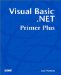Visual Basic .NET Primer Plus
ISBN: 0672324857
EAN: 2147483647
Year: 2003
Pages: 238
Authors: Jack Purdum

Similar book on Amazon